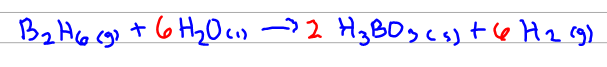# Problem: Calculate the mass (g) of each product formed when 43.82 g of diborane (B 2H6) reacts with excess water: B2H6(g) + H2O(l) ⟶H3BO3(s) + H2(g) [unbalanced]

🤓 Based on our data, we think this question is relevant for Professor Powe's class at UOFL.

###### FREE Expert Solution

First, get the balanced reaction :###### Problem Details

Calculate the mass (g) of each product formed when 43.82 g of diborane (B 2H6) reacts with excess water: B2H6(g) + H2O(l) ⟶H3BO3(s) + H2(g) [unbalanced]